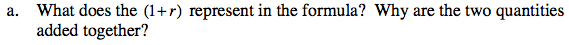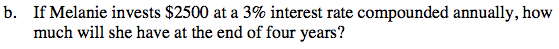Home > ACC7 > Chapter cc38 > Lesson cc38.1.3 > Problem8-30

8-30.
1. Read this lesson’s Math Notes box about compound interest, and then answer the questions below. Homework Help ✎

1. What does the (1 + r) represent in the formula? Why are the two quantities added together?

2. If Melanie invests $2500 at a 3% interest rate compounded annually, how much will she have at the end of four years?Multiplying the principal amount by 1 results in the original amount. Multiplying the principal by the interest rate results in the amount gained from interest. These values are added to calculate the percent based on the previous balance, not just the principal.$\text{Use}\; {\it A} = {\it P}(1 + {\it r})^{\it n}.$ Where P is principal, r is the interest rate, and n is time. $(2500)(1+0.03)^{4}$ A =$2813.77